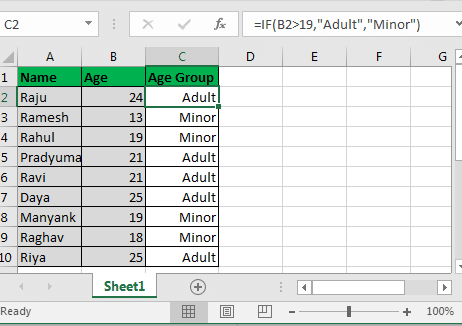# = IF (condition, value if TRUE, value if FALSE)

The IF statement in Excel checks the condition and returns the specified value for each of the answers if it is true ( TRUE ) or false ( FALSE ). In the value if TRUE and value if FALSE sections , you can enter a value, text with quotes, another formula, or even another if (use the nested IF statement ).

Note: In Excel 2016 , 2013 and 2010 you can have a maximum of 64 nested if statements , but in Excel 2007 you can only have 7 nested ifs .

The best thing about the IF phrase is that you can customize the TRUE and FALSE results and show anything in the output. And that’s exactly what we need. Let’s see how we can do this…

#### Example 1 for the if statement:

Suppose you have a list of people. Now just suppose you want to know how many of them are adults and how many of them are minors.

We consider that people over the age of 19 are adults and we consider those under the age of 19 to be under the legal age.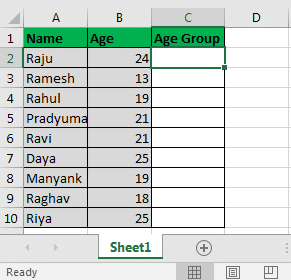In cell C2, write this conditional statement and drag it down:

= IF (B2> 19, “Adult”, “Minor”)

Here Excel software simply checks if the B2 cell value is greater than 19. Since it is more than 19, it shows adult in C2 . And it does the same for the rest of the cells. Finally, the result of our work is the image below:This is a simple example of how the IF function works in Excel. However, most of the time you need a nested IF or a combination of the if function with other functions.

Let’s look at another example of an IF function .

#### Example 2: Nested ifs to examine multiple conditions

Suppose in a specific list, you must specify whether the student is an adult or a “teen or child.” And if he is young, he can be said to be a teenager (between 13 and 19 years old) or a child (under 13 years old).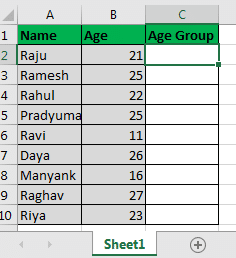So here’s what we need to do:

We must specify if the age of the students is <20, check whether the age of the students is <13, if the answer is yes then the student is ” KID ” (child), if not, indicate ” Teenager ” (adolescent), and If the answer to the first question is no, it shows adult .

There are other ways to do this, but we will look at this example together to better understand.

Information: In most functions, the answers are calculated outside the expression, but not in the if expression .

In a nested IF function , first the external IF and then the internal IF are calculated.

The image below is a basic diagram of the nested IF control flow .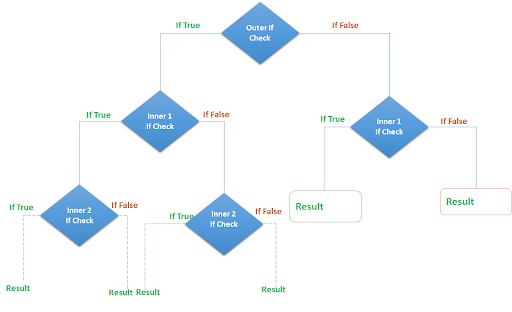In cell C2 , write this formula IF and drag it to cell C10 :

= IF (B6 <20, IF (B6 <13, “KID”, “Teenager”), “Adult”)

The result of our work will look like the image below: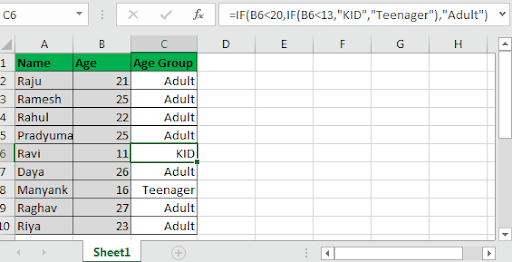Isn’t it easy?

If B6 > 20: The conditional statement checks for this.

Since this statement is correct, it goes to the next condition and checks whether B6 is <13 or not. If the answer is TRUE , it sets the value to ” KID ” and if it is not, it sets the value to ” Teenager “. If the answer to both conditions is FALSE , it sets the value to adult .

Since in this case more than 20 years of age or equal to 20, as adult (adult) are shown.

Note that Ravi as ” KID ” (child) displayed because of his age is 11 years old and Manyank at age 16 as a ” Teenager ” (Teen) will be shown.